#### How to Make Ogive Chart in Excel

It is a pity that the ogive chart is not a defined chart type in Excel. I sometimes use it and for this reason I have to do an ogive graph step by step. Below I will show you how to easily create an ogive chart in Excel.

## What is Ogive Chart

An ogive plot allows you to show the cumulative frequency of data values. Therefore, the cumulative frequency plot is always an ascending line that grows by leaps and bounds as you cross data boundaries.

When inserting an Ogive (Cumulative Frequency) chart, the first thing you need is data like this: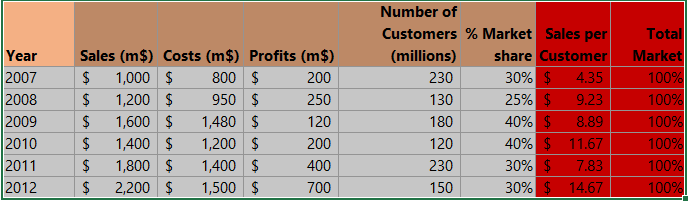As you can see in the picture above, the key step is to properly prepare the data table. When creating an ogive chart, you are faced with a difficult task.

The most difficult thing to do is to define the data ranges so that they do not disturb the frequency graph. If possible, try to keep the data intervals of the same size. Actually, it is from the point of view of the cumulative sum graph, so that they are of equal width.

In my example, I decided to create age brackets 15 years wide. Only the first compartment is of a different width. It is allowed that the first and last bins have a different width. It is all the more acceptable when the data values in these ranges are small.

Write ages on an empty column (1), and write the last numbers in the ages (2).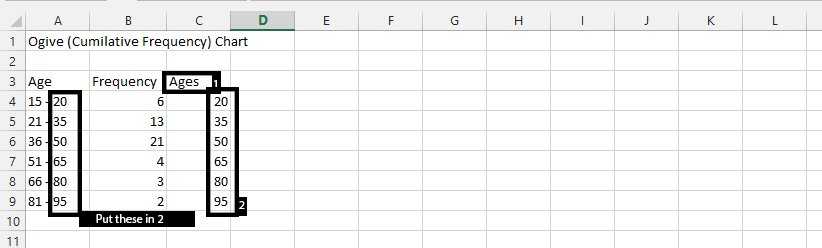## Inserting a cumulative frequency

In column C, enter the upper ranges of the previously defined ranges. As I wrote earlier, it is 20 for me and each subsequent one is 15 more.

Remember that the task of the ogive chart is to show the sum of the accumulated data? In an empty column, write Cumulative Frequency and proceed to calculate the cumulative sum in column D below.

Click under the Cumulative Frequency, and type =B4, which is the value under Frequency in column B.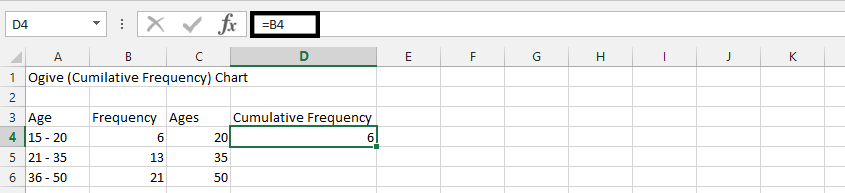Click under the result from the previous result (1) and type =B5+B4.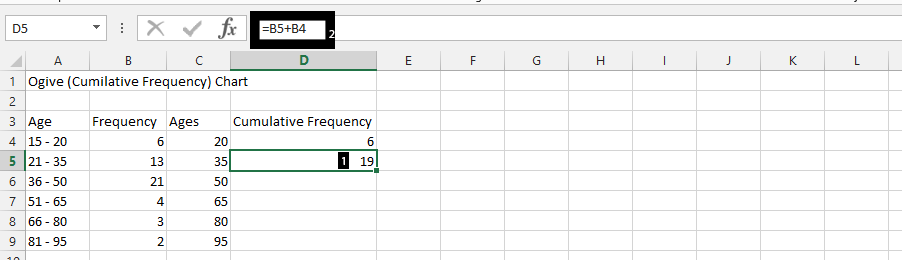Note: Follow this step on the rest, by typing =SUM(B6: B4 beside 50, =SUM(B7:B4) beside 65, =SUM(B8:B4) beside 80, and =SUM(B9:B4) beside 95.

Choose two cells under ages and cumulative frequency, right-click on them (1), and choose insert (2).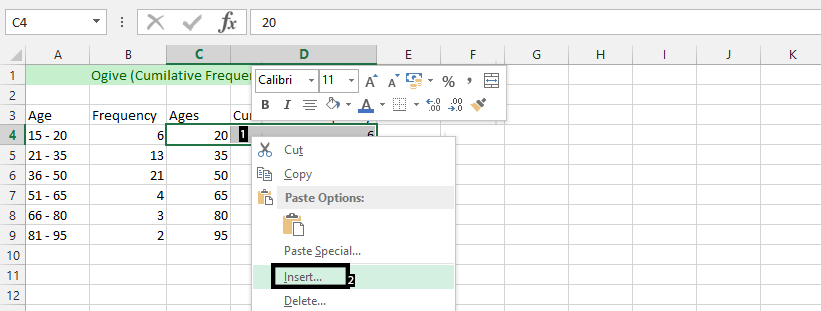Choose to shift cells down (1), and press OK (2).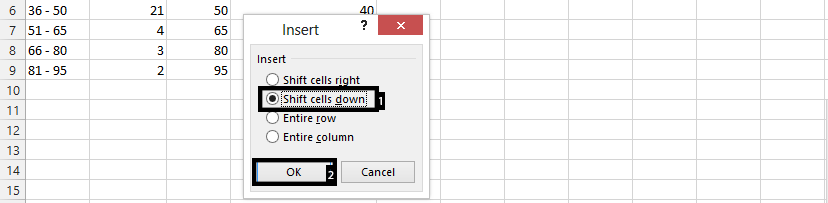Put 0 under the cumulative frequency (1), and choose a number that rhymes with the ages under ages (2).

Highlight all of the rows in terms of both ages and cumulative frequency.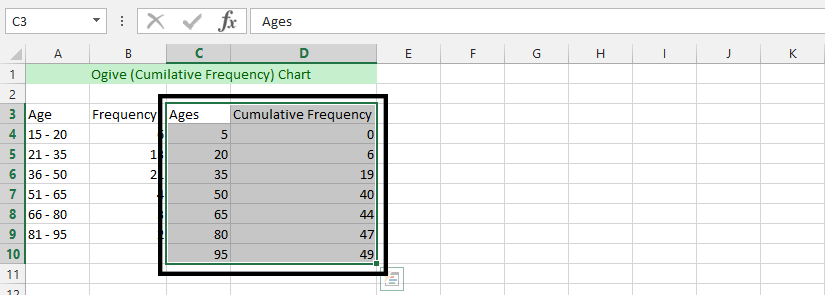## Inserting an ogive chart

Having calculated the cumulative frequency of your data, you can start creating a cumulative chart.

Ogive charts are usually scatter charts, but you can also choose to use a line chart. The important thing is that the plot should contain both a point and a line. I chose the scatter plot.

Select insert (1), scatter chart (2), and scatter chart with marker (3).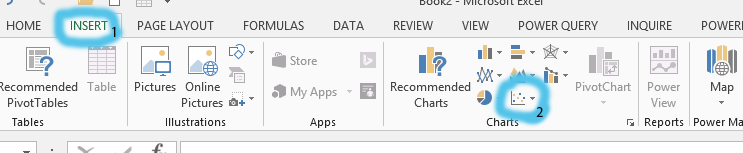In conclusion, we have created an ogive chart that looks like this: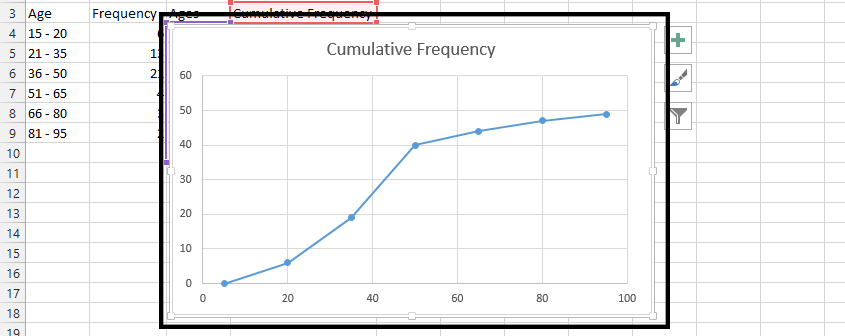You can clearly see the value line that grows as the data ranges increase. At first glance, you can see that the greatest increase occurred between 35 and 50.

## Formatting an ogive chart

At the moment, the ogive graph is ready, but you can think about further improving your graph.

The first improvement is the adjustment of the X axis to the data you present on the chart. As you can see in my example, the axis scale does not match the data.

In this case, I suggest you go to Format Axis and change 3 things:

• Minimum Bound – set as the beginning of the first range of data in your data table, i.e. the theoretically lowest value
• Maximum Bound – it will be the end of the last range, i.e. the highest value, respectively
• Major Unit – the width of the data range, i.e. if the age increases by 15 years, enter 15.

The last of the improvements that I recommend you to change is the change of gridlines. As reading an ogive chart relies on identifying points, increasing the number of horizontal and vertical lines in the chart will be of great help. This way, the person reading your graph will be able to assign values to points.

At this link, you will learn how to add a grid in Excel.

If you don’t like adding gridlines then alternatively you can add data labels. The exact values from the data table appear next to the points on the graph.

You can further customize the chart to your liking by changing the chart title, axis labels, font styles, and colors.

## Interpreting an ogive chart

Interpreting an Ogive chart in Excel involves understanding what the graph is trying to convey and how to use that information to make conclusions about the data.

Here are some steps to interpret an Ogive chart in Excel:

1. Determine the cumulative frequency: The Y-axis of the Ogive chart represents the cumulative frequency, which is the sum of the frequencies of all data points up to a certain point.
2. Identify the data points: The X-axis of the Ogive chart represents the data set, which is the set of values that you are analyzing.
3. Look at the shape of the graph: The shape of the Ogive chart can help you to understand the distribution of the data. If the graph is roughly symmetrical, then the data is likely to be normally distributed. If the graph is skewed to one side or the other, then the data is likely to be skewed in that direction.
4. Use the graph to find specific cumulative frequencies: By looking at the graph, you can easily determine the cumulative frequency of any data point. For example, if you want to find the cumulative frequency of a data point with a value of 50, you would look for the point on the graph where the X-axis intersects the 50 value, and then look at the corresponding value on the Y-axis to determine the cumulative frequency.
5. Make conclusions about the data: By using the information from the Ogive chart, you can make conclusions about the data set. For example, you can determine the percentage of data points that fall within a certain range, or you can find the median value of the data set.